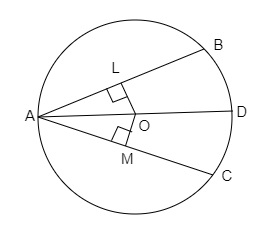# If two chords of a circle are equally inclined to the diameter through their point of intersection, prove the chords are equal.

Given:

Two chords of a circle are equally inclined to the diameter through their point of intersection.

To do:

We have to prove that the chords are equal.
Solution:$AB$ and $AC$ are two chords which are equally inclined to the diameter $AD$ of the circle with centre $O$.
Draw $OL \perp AB$ and $OM \perp AC$.

In $\triangle OLA$ and $\triangle OMA$,

$\angle OLA=\angle OMA=90^o$
$OA=OA$    (Common side)
$\angle LAO=\angle MAO$   (Given)

Therefore,
$\triangle \mathrm{OLA} \cong \triangle \mathrm{OMA}$ (By AAS congruence)
$\Rightarrow \mathrm{OL}=\mathrm{OM}$       (CPCT)
This implies, the chords are equidstant from the centre $O$.
$\Rightarrow A B=A C$     (Equal chords of a circle are equidistant from the centre of the circle)
Hence proved.

Updated on: 10-Oct-2022

87 Views